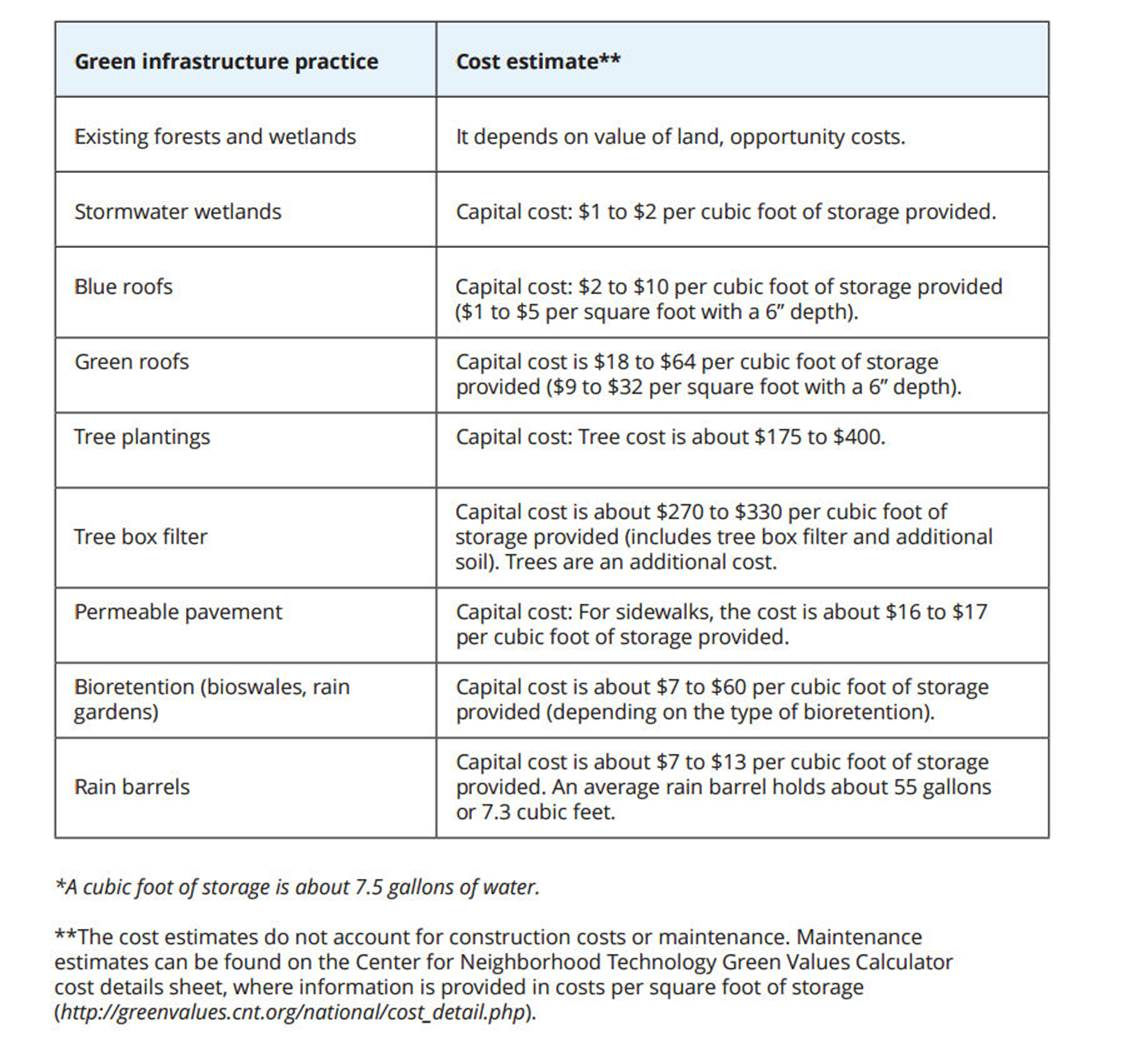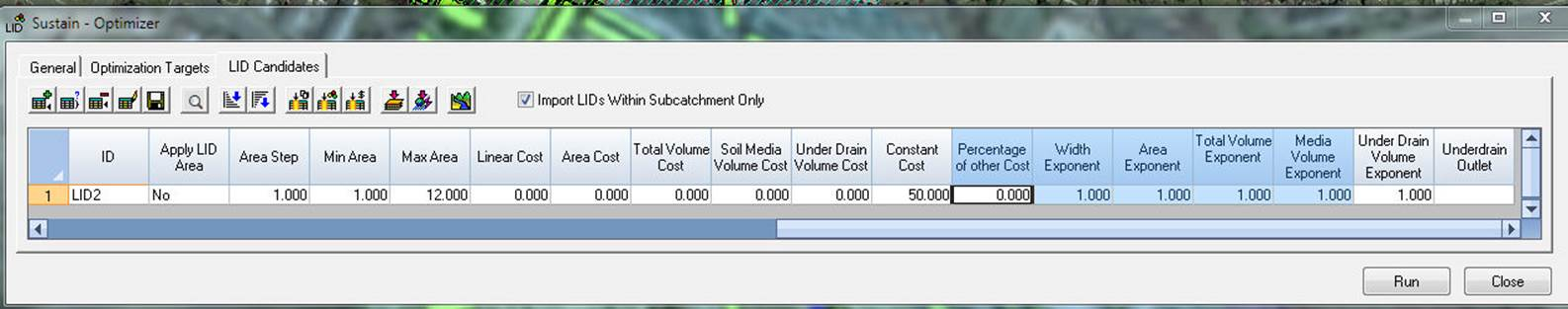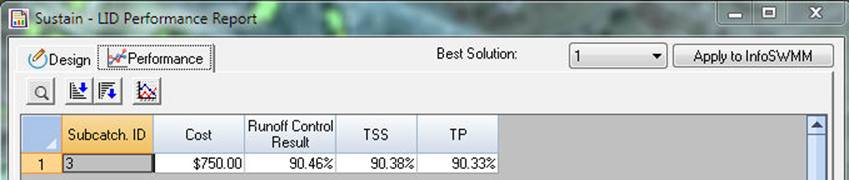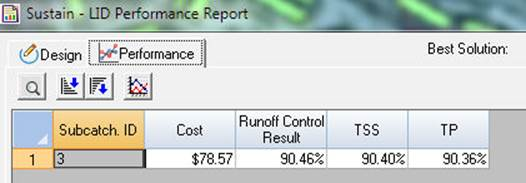#INFOSWMM

# Tutorial 13 How are the Costs Used in the LID Candidate Table for InfoSWMM Sustain?

There are seven type of costs associated with a LID or SuDS in InfoSWMM Sustain as shown in the following table.

 Type of Cost Description of Cost Linear Cost Cost per linear unit of the LID or SuDs or \$/foot or \$/meter. Linear is Unit Area / Unit Width Area Cost Cost per area of the LID \$/foot^2 or \$/meter^2 Total Volume Cost Cost per volume of the LID \$/foot^3or \$/meter^3 Soil Media Volume Cost Cost per volume of the LID \$/foot^3or \$/meter^3 Under Drain Volume Cost Cost per volume of the LID \$/foot^3or \$/meter^3 Constant Cost Constant Cost per Unit or Replicate Percentage of other Costs Percentage of the Other Costs or Overhead/Multiplier Costs

#### A Good Estimate of LID or SuDS Costs

Green infrastructure practice Cost estimate** Existing forests and wetlands It depends on value of land, opportunity costs. Stormwater wetlands Capital cost: \$1 to \$2 per cubic foot of storage provided. Blue roofs Capital cost: \$2 to \$10 per cubic foot of storage provided (\$1 to \$5 per square foot with a 6” depth). Green roofs Capital cost is \$18 to \$64 per cubic foot of storage provided (\$9 to \$32 per square foot with a 6’’ depth). Tree plantings Capital cost: Tree cost is about \$175 to \$400. Tree box filter Capital cost is about \$270 to \$330 per cubic foot of storage provided (includes tree box filter and additional soil). Trees are an additional cost. Permeable pavement Capital cost: For sidewalks, the cost is about \$16 to \$17 per cubic foot of storage provided. Bioretention (bioswales, rain gardens) Capital cost is about \$7 to \$60 per cubic foot of storage provided (depending on the type of bioretention). Rain barrels Capital cost is about \$7 to \$13 per cubic foot of storage provided.#### Cost are part of the Objective Function#### Cost Example 1 – Constant Cost

In this example we have a constant cost of \$50 per Rain Barrel The optimum solution is 10 Rain Barrels or a total cost of \$500.#### Cost Example 2 – Constant Cost + Percentage of Other Cost

In this example we have a constant cost of \$50 per Rain Barrel + 50 percent of other cost. The optimum solution is 10 Rain Barrels or a total cost of \$750.#### Cost Example 3 – Constant Cost + Linear Cost

In this example we have a constant cost of \$50 per Rain Barrel + \$10 – Linear Cost. We will change the area and width as we as now optimizing Cisterns. The optimum solution is 10 Cisterns or a total cost of \$800 or 10 * \$50 + 10*\$3*\$10 The linear unit is 12 feet^2/4 feet width

#### Cost Example 4 – Area Cost

In this example we have a constant cost of \$0 per Rain Barrel + \$10 Area Cost. We will change the area and width as we as now optimizing Cisterns. The optimum solution is 10 Cisterns or a total cost of \$78.57

For a RB or Cistern the area of the LID is BMParea = 3.142857/4.0*length^2).#### Cost Example 5 – Volume Cost

In this example we have a constant cost of \$0 per Rain Barrel + \$10 Volume Cost. We will change the area and width as we as now optimizing Cisterns. The optimum solution is 10 Cisterns or a total cost of \$314.29#### Cost Example 7 – Underdrain Volume Cost

In this example we have a constant cost of \$0 per Rain Barrel + \$10 Under Drain Volume Cost. We will change the area and width as we as now optimizing Cisterns. The optimum solution is 10 Cisterns or a total cost of \$314.29